# Area + sphere - math problems

#### Number of problems found: 45

• Sphere areaA cube with edge 1 m long is circumscribed sphere (vertices of the cube lies on the surface of a sphere). Determine the surface area of the sphere.
• Sphere cutsAt what distance from the center intersects sphere with radius R = 56 plane, if the cut area and area of the main sphere circle is in ratio 1/2.
• Sphere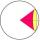Intersect between plane and a sphere is a circle with a radius of 60 mm. Cone whose base is this circle and whose apex is at the center of the sphere has a height of 34 mm. Calculate the surface area and volume of a sphere.
• Cube and sphereCube with the surface area 150 cm2 is described sphere. What is sphere surface?The radius of the sphere we reduce by 1/3 of the original radius. How much percent does the volume and surface of the sphere change?
• Sphere vs cubeHow many % of the surface of a sphere of radius 12 cm is the surface of a cube inscribed in this sphere?
• Sphere VSFind the surface and volume of a sphere that has a radius of 2 dm.
• Cube from sphere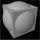What largest surface area (in cm2) can have a cube that was cut out of a sphere with radius 43 cm?
• Sphere A2VThe surface of the sphere is 241 mm2. What is its volume?
• Sphere - partsCalculate the area of a spherical cap, which is part of an area with base radius ρ = 9 cm and a height v = 3.1 cm.
• Spheres in sphere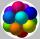How many spheres with a radius of 15 cm can fit into the larger sphere with a radius of 150 cm?
• Iron sphereIron sphere has weight 100 kg and density ρ = 7600 kg/m3. Calculate the volume, surface and diameter of the sphere.The surface of the sphere is 60 cm square. Calculate its radius; result round to tenth of cm.
• Half-sphere roofThe roof above the castle tower has the shape of a 12.8 m diameter half-sphere. What is the cost of this roof, if the cost of 1 square meter is 12 euros and 40 cents?
• SphereThe surface of the sphere is 12100 cm2, and the weight is 136 kg. What is its density?
• Equilateral cylinder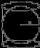A sphere is inserted into the rotating equilateral cylinder (touching the bases and the shell). Prove that the cylinder has both a volume and a surface half larger than an inscribed sphere.
• A spherical segment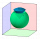A spherical section whose axial section has an angle of j = 120° in the center of the sphere is part of a sphere with a radius r = 10 cm. Calculate the cut surface.
• Sphere slicesCalculate the volume and surface of a sphere if the radii of parallel cut r1=31 cm, r2=92 cm, and its distance v=25 cm.
• The volumeThe volume of the sphere is 1 m3, what is its surface?How many times does the surface of a sphere decrease if we reduce its radius twice?